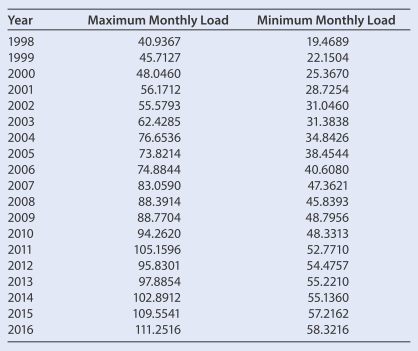### Mathematical Applications for the ...

11th Edition
Ronald J. Harshbarger + 1 other
Publisher: Cengage Learning
ISBN: 9781305108042

#### Solutions

Chapter
Section### Mathematical Applications for the ...

11th Edition
Ronald J. Harshbarger + 1 other
Publisher: Cengage Learning
ISBN: 9781305108042
Chapter 13, Problem 1EAGP1
Textbook Problem
1 views

## I. Purchasing Electrical Power (Modeling)In order to plan its purchases of electrical power from suppliers over the next 5 years, the PAC Electric Company needs to model its load data (demand for power by its customers) and use this model to predict future loads. The company pays for the electrical power each month on the basis of the peak load (demand) at any point during the month. The table gives, for the years 1998–2016, the load in megawatts (that is, in millions of watts) for the month when the maximum load occurred and the load in megawatts for the month when the minimum load occurred. The maximum loads occurred in summer, and the minimum loads occurred in spring or fall.The company wishes to predict the average monthly load over the next 5 years so that it can plan its future monthly purchases. To assist the company, proceed as follows. Using the years and the maximum monthly load given for each year, graph the data, with x representing the number of years past 1998 and y representing the maximum load in megawatts. Find the equation that best fits the data, using both a quadratic model and a cubic model. Graph the data and both of these models from 1998 to 2018 (that is, from x = 0 to x = 20 ).

This textbook solution is under construction.

### Still sussing out bartleby?

Check out a sample textbook solution.

See a sample solution

#### The Solution to Your Study Problems

Bartleby provides explanations to thousands of textbook problems written by our experts, many with advanced degrees!

Get Started